milk>試卷(2021/11/26)

110 年 - 110 國立中山大學碩士暨碩士專班招生考試_部分碩士班:電子學#104281

【非選題】
1.

1.(15%) A second-order filter has its poles at s = -(1/4)The transmission is zero atω = 3 rad/s and is unity at dc (ω = 0). Find the transfer function. (15%*1)

【非選題】
2.

2. (20%) In the circuit of Fig. 1, the NMOS transistor has|Vt| = 0.9 V, and VA = 50 V, and operates with VD =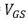= 2 V.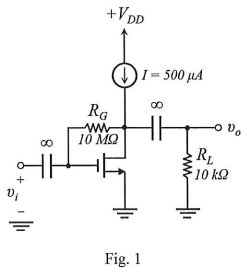【題組】 (a) What is the voltage gain vo/vi ? (10%)

【非選題】
3.【題組】 (b) What do VD and

【非選題】
4.【題組】(c) the gain become for I increased to 1 mA? (5%*2)

【非選題】
5.

3.(30%) For the common-base circuit in Fig. 2, assuming the bias current to be about 1 mA, β = 100, Cμ = 0.8 pF, re = 25Ω, and fT = 500 MHz: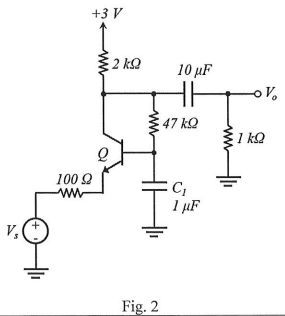【題組】(a) Estimate the midband gain Vo/Vs.

【非選題】
6.【題組】

(b) Use the short-circuit time-constants method to estimate the lower 3-dB frequency, fL. (Hint: In determining the resistance seen by C1, the effect of the 47-kΩ resistor must be taken into account.)

【非選題】
7.【題組】(c) Find the high-frequency poles, and estimate the upper 3-dB frequency, fH. (10%*3)

【非選題】
8.

4.(35%) The amplifier of Fig. 3 consists of two identical common-emitter amplifiers connected in cascade. Observe that the input resistance of the second stage,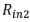, constitutes the load resistance of the first stage.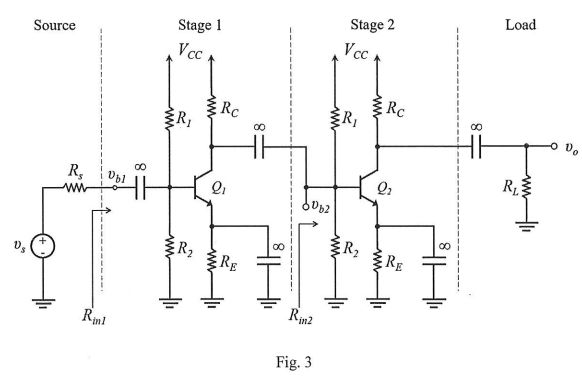【題組】(a) For= 15 V,VT = 0.025 V, R1 = 100 kΩ, R2= 47 KΩ, RE = 3.9 kΩ, Rc= 6.8 kΩ, and β = 100, determine the de collector current of each transistor. (5%)

【非選題】
9.【題組】

(b) Draw the small-signal equivalent circuit of the entire amplifier and give the values of all its components. Neglect.(10%)

【非選題】
10.【題組】

(c) Find【非選題】
11.【題組】

(d) Find【非選題】
12.【題組】

(e) For RL= 2 kΩ, find.

【非選題】
13.【題組】(f) Find the overall voltage gain vo/vs. (5%*4)

懸賞詳解

國三數學上第一次

1.下圖為三角形紙片ABC，其中D點和E點將 分成三等分，F點為DE中點。若小慕從AB上的一點P，沿著與直線 平行的方向將紙片剪開後，剪下的小三角形紙片�...